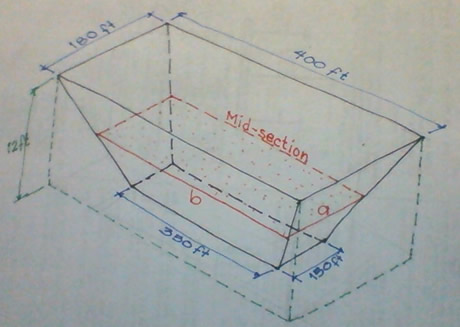# MATHalino 2020 (Ito Yung Last sa Paatras) Solid mensuration problem

2 posts / 0 new
miich
MATHalino 2020 (Ito Yung Last sa Paatras) Solid mensuration problem

1)An excavation is 12 ft. deep and has trapezoidal sides (faces). The upper base is horizontal rectangle 400 ft. by 180 ft., and the lower base is a horizontal rectangle 350 ft. by 150 ft. How many cubic yards of earth were removed in digging the excavation?

2)A chimney in the shape of a frustum of a regular pyramid is 186.3 ft. high. Its upper base is a square 10 ft. on a side, and its lower base is a square 16 ft. on a side. The flue is of uniform square cross section. 7 ¼ by 7 ¼ ft. find the weight of the chimney if the material weighs 112.8 lb. per cu. ft.

Jhun VertNumber (1)
Upper base area
$A_1 = 400 \times 180 = 72\,000 ~ \text{ft}^2$

Lower base area
$A_2 = 350 \times 150 = 25\,500 ~ \text{ft}^2$Mid section
$a = \frac{1}{2}(180 + 150) = 165 ~ \text{ft}$

$b = \frac{1}{2}(400 + 350) = 375 ~ \text{ft}$

$A_m = ab = 165 \times 375 = 61\,875 ~ \text{ft}^2$

Distance between A1 and A2
$L = 12 ~ \text{ft}$

Volume of excavation (Prismatoid)
$V = \frac{1}{6}L(A_1 + 4A_m + A_2)$

$V = \frac{1}{6}(12) [ \, 72\,000 + 4(61\,875) + 25\,500 \, ]$

$V = 690\,000 ~ \text{ft}^3$

$V = 690\,000 ~ \text{ft}^3 \left( \dfrac{1 ~ \text{yd}}{3 ~ \text{ft}} \right)^3$

$V = 25\,555.56 ~ \text{yd}^3$

• Mathematics inside the configured delimiters is rendered by MathJax. The default math delimiters are $$...$$ and $...$ for displayed mathematics, and $...$ and $...$ for in-line mathematics.